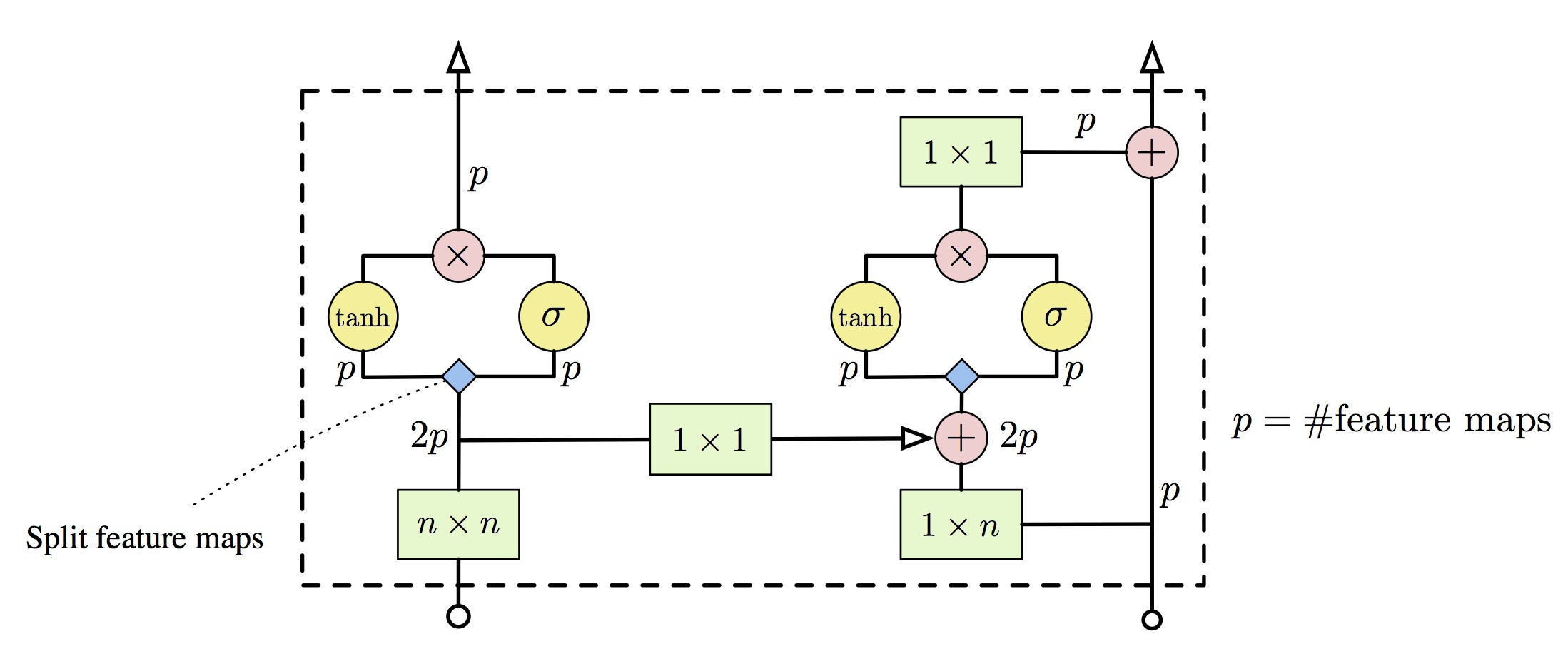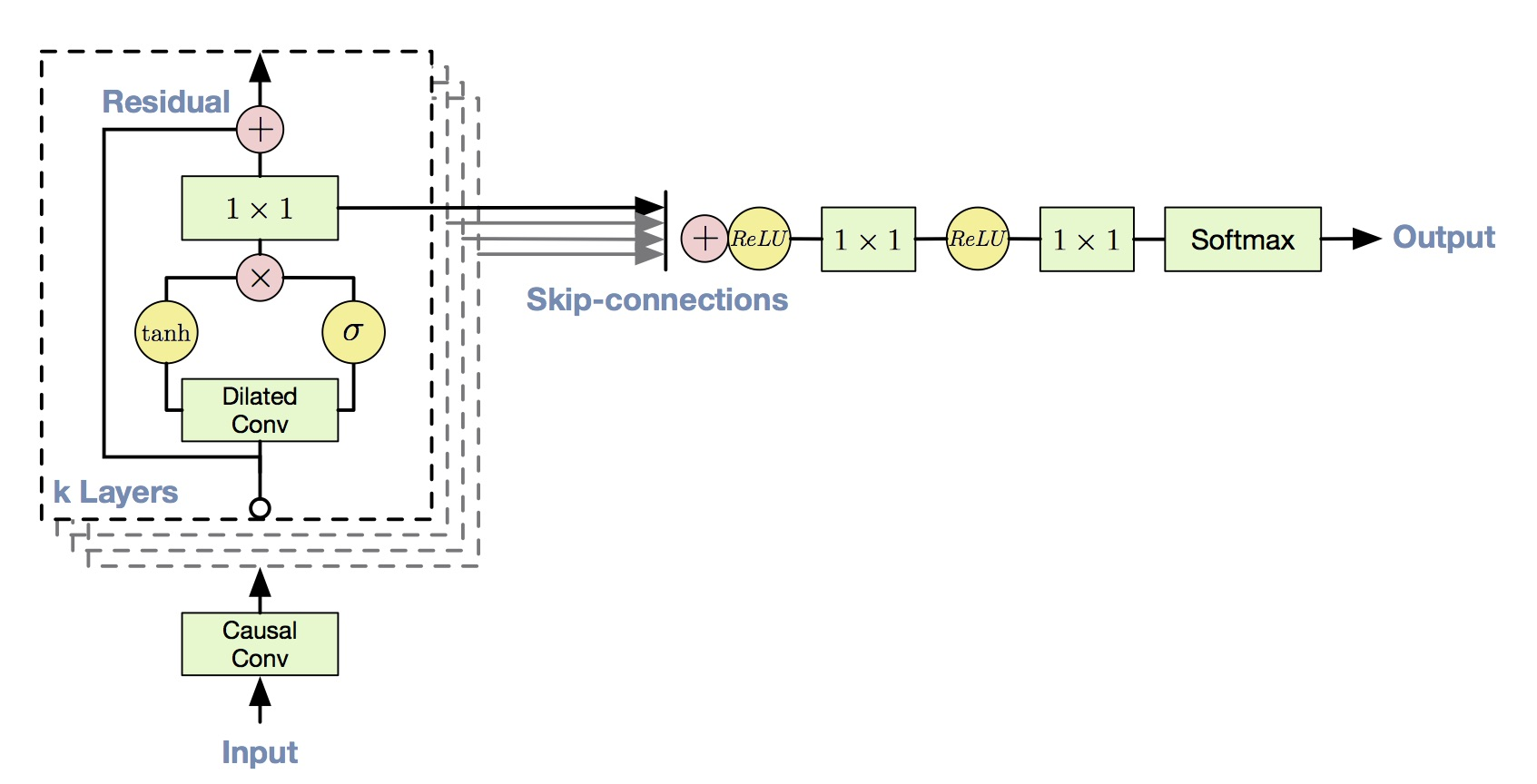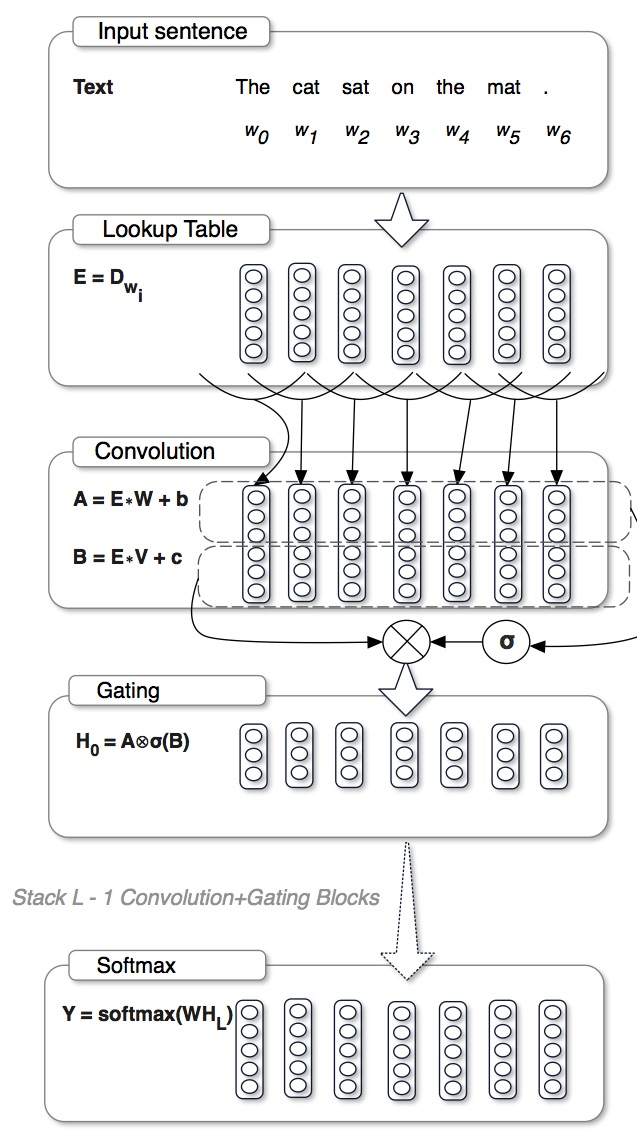This is a brief note for the three papers in the title, Pixel CNN (specifically their nips paper), Wavenet, Language modeling with GCNN.

These three enjoy a similar gate-based structure and are all an autoregressive model for generation (of images, audios and language). This kind of autoregressive model is very common in language modeling, where people commonly use LSTM to predict the next word given all the previous words. Now it’s applied to more area.

Pixel CNN and Wavenet are from Deepmind, and GCNN is from FAIR.

### Pixel CNN

The Pixel CNN paper is based their earlier paper Pixel RNN. Pixel RNN is exactly to apply the language modeling method on images. Since the 2D structure of images, they use grid LSTM. (They also propose a simple pixel CNN in the pixel RNN paper, but that model has blind spot, aka. not using all the previous pixel))

The conditional pixel CNN (their nips paper) propose a better pixel CNN, which doesn’t have blind spot any more. They propose two convnet stacks: horizontal and vertical. The vertical stacks see all the pixel above (not including current row, the blue parts in the following image), and the horizontal stacks see all the pixels left in the row (purple area in the following image).Vertical and Horizontal Stacks

Note that, the features in vertical stacks are fused in horizontal stacks, so the horizontal can actually also see the pixels above. And they use residual in horizontal but no residual in vertical.

To avoid seeing future pixels, they use the masked convolution. In addition, they use gated activation unit, the formula is like this:

${\bf y} = \tanh(W_f * {\bf x}) \odot \sigma(W_g * {\bf x})$

They borrow this activation from LSTM, highway network and neural GPU, and claim better performace. The following image shows the actual block in the network.A single layer in the Gated PixelCNN architecture. Convolution operations are shown in green, element-wise multiplications and additions are shown in red. The convolutions with W_f and W_g are combined into a single operation shown in blue, which splits the 2p features maps into two groups of p

By adding another input to the gated unit, they can get conditional pixel CNN (the additional input can be location-awared or not). The experiements shows they can get the best average Negative Log-Likelihood per pixel on several datasets.

The pros of Pixel CNN compared to GAN:

• Provides a way to calculate likelihood. (A reasonable metric)

• the training is more stable than GANs.

• Works for both discrete and continuous data.

The cons:

• The model assumes the order of generation: top to down, left to right. (Doesn’t really make sense)

• Slow in sampling.

• Slow in training: PixelCNN++ (from OpenAI) converges in 5 days on 8 Titan for CIFAR dataset. (however faster than pixel RNN).

• Worse sample quality.

• Haven’t been applied for feature learning.

(The pros and cons are tranlated from How to compare between PixelCNN and DCGAN, credit on Xun Huang)

### Wavenet

The wavenet is very similar to pixelCNN in structure, but is much more successful in genrating audio than PixelCNN in image generation. The model is trained on raw audio waveforms and can generate raw audios.

To vastly increase the receptive field, they use dilated convolution, which can have much larger receptive field with the same number of parameters than normal convolution.Dilated causal convolutional layers

In their final structure, they actually stack a bunch of layers like this image together.

They use classification framework instead regression to generate the next sample point. The continuous audio is quantitized into 256 values.

They also use residual and gated activation here just as in pixel CNN. In addition, they also use skip connection.The entire architecture

Similarly, they could also make this model conditional by adding another input to the gated unit. The conditional input could be a one-hot vector of identity of the speaker or linguistic features of texts for TTS.

Although they use dilated convolution to expand the receptive field, the receptive field is only about 300 milliseconds. So they also add another module called context stacks, which can work in a low resolution and can see more previous signals with few parameters.

The results of wavenet is very remarkable; you can find examples here.

The wavenet was known as slow in generation, but a recent paper proposes a simple way to accelerate it. The idea of algorithm is just to remove some redundant computations (think of brute search to dynamic programming). (Although the algorithm is very useful, the author list is too long for this amount of work.)

Similar to PixelCNN, the training is also slow.

### Gated CNN

I put GCNN here because it also has the gate structure, making me curious about why this kind of structure suddenly becomes so popular.

The gated unit is slightly different from that in previous two. By throwing away the $\tanh$, the so-called Gated Linear Unit is:

${\bf y} = (W_1 * {\bf x}) \odot \sigma(W_2 * {\bf x})$

They claim this kind of unit can suffer less from the gradient vanishing problem.GCNN

This paper is very good because they provides a detailed ablation study.

• With respect to the final perplexity on WikiText-103, Gated Linear Unit is the best, and ReLU and GTU (Gated Tanh Unit, what’s used in pixelCNN and wavenet) work similar, and Tanh is the worst.

• From the comparison between GLU and GTU, we know removing the $\tanh$ is better.

• From the comparison between GTU and Tanh, we know that the sigmoid gating is essential.

• From the comparison between GLU and ReLU, we know that the learnable gating function is essential(You can think of ReLU as a special case of GLU, where the gate function is $({\bf X} > 0)$).

• They also analyze the the nonlinearity. They compare linear (no gate), blinear (gate is a linear function) and GLU, and GLU is the best.

• Depth: good if the depth is less than the sentence length. (Link depth to length is a little weird to me)

• context size: preformance diminishes quickly after context of 20 words, which is congruent to the fact that the RNN can achieve good performace even after truncating the gradients after 20 steps.

• The usage of weight normalization and gradient clipping help alot

### Conclusion

It seems that gate structure is recently quite popular in generation task. Although highway network which is the first to apply LSTM-like structure to CNN doesn’t work well, the recent applications of gated unit can achieve the state-of-art result. It’s interesting to investigate why this gate is so useful.